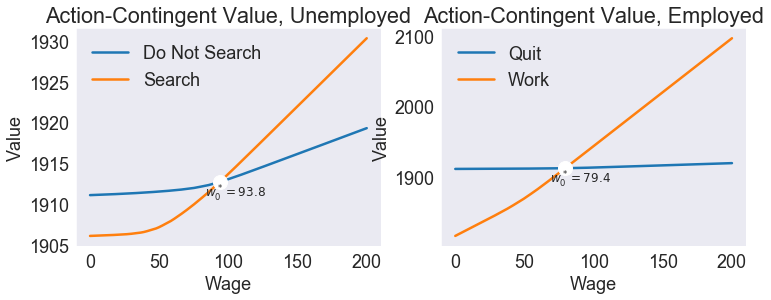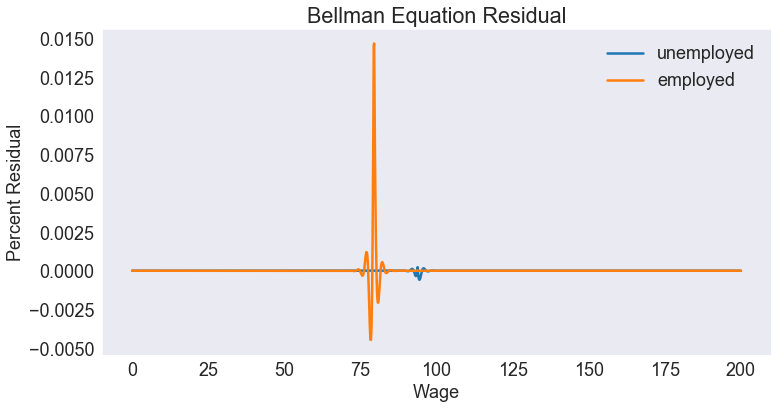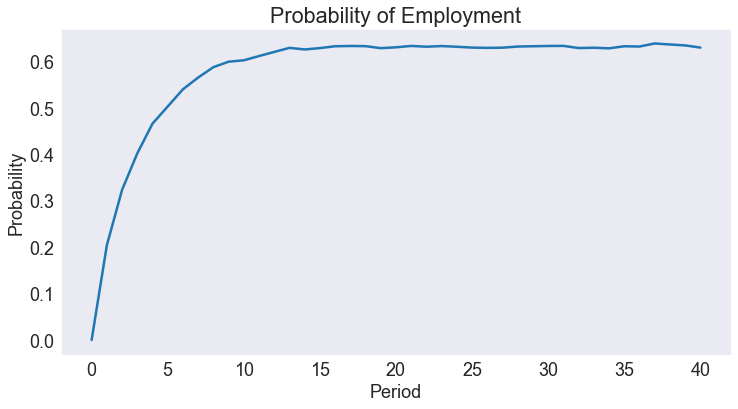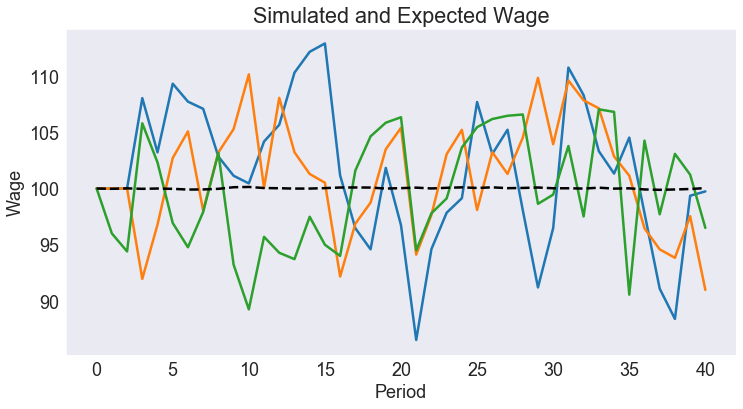# Job Search Model¶

Infinitely-lived worker must decide whether to quit, if employed, or search for a job, if unemployed, given prevailing market wages.

### States¶

• w prevailing wage
• i unemployed (0) or employed (1) at beginning of period

### Actions¶

• j idle (0) or active (i.e., work or search) (1) this period

### Parameters¶

Parameter Meaning
$v$ benefit of pure leisure
$\bar{w}$ long-run mean wage
$\gamma$ wage reversion rate
$p_0$ probability of finding job
$p_1$ probability of keeping job
$\sigma$ standard deviation of wage shock
$\delta$ discount factor

In :
import numpy as np
import pandas as pd
import matplotlib.pyplot as plt
from compecon import BasisSpline, DPmodel, qnwnorm, demo


## FORMULATION¶

### Worker's reward¶

The worker's reward is:

• $w$ (the prevailing wage rate), if he's employed and active (working)
• $u=90$, if he's unemployed but active (searching)
• $v=95$, if he's idle (quit if employed, not searching if unemployed)
In :
u     =  90
v     =  95

def reward(w, x, employed, active):
if active:
return w.copy() if employed else np.full_like(w, u)  # the copy is critical!!! otherwise it passes a pointer to w!!
else:
return np.full_like(w, v)


### Model dynamics¶

#### Stochastic Discrete State Transition Probabilities¶

An unemployed worker who is searching for a job has a probability $p_0=0.2$ of finding it, while an employed worker who doesn't want to quit his job has a probability $p_1 = 0.9$ of keeping it. An idle worker (someone who quits or doesn't search for a job) will definitely be unemployed next period. Thus, the transition probabilities are \begin{align} q = \begin{bmatrix}1-p_0 &p_0\\1-p_1&p_1\end{bmatrix},&\qquad\text{if active} \\ = \begin{bmatrix}1 & 0\\1 &0 \end{bmatrix},&\qquad\text{if iddle} \end{align}

In :
p0    = 0.20
p1    = 0.90

q = np.zeros((2, 2, 2))
q[1, 0, 1] = p0
q[1, 1, 1] = p1
q[:, :, 0] = 1 - q[:, :, 1]


#### Stochastic Continuous State Transition¶

Assuming that the wage rate $w$ follows an exogenous Markov process \begin{equation} w_{t+1} = \bar{w} + \gamma(w_t − \bar{w}) + \epsilon_{t+1} \end{equation}

where $\bar{w}=100$ and $\gamma=0.4$.

In :
wbar  = 100
gamma = 0.40
def transition(w, x, i, j, in_, e):
return wbar + gamma * (w - wbar) + e


Here, $\epsilon$ is normal $(0,\sigma^2)$ wage shock, where $\sigma=5$. We discretize this distribution with the function qnwnorm.

In :
sigma = 5
m = 15
e, w = qnwnorm(m, 0, sigma ** 2)


### Approximation Structure¶

To discretize the continuous state variable, we use a cubic spline basis with $n=150$ nodes between $w_\min=0$ and $w_\max=200$.

In :
n = 150
wmin = 0
wmax = 200
basis = BasisSpline(n, wmin, wmax, labels=['wage'])


## SOLUTION¶

To represent the model, we create an instance of DPmodel. Here, we assume a discout factor of $\delta=0.95$.

In :
model = DPmodel(basis, reward, transition,
i =['unemployed', 'employed'],
j = ['idle', 'active'],
discount=0.95, e=e, w=w, q=q)


Then, we call the method solve to solve the Bellman equation

In :
S = model.solve(show=True)

Solving infinite-horizon model collocation equation by Newton's method
iter change       time
------------------------------
0       2.1e+03    0.2739
1       3.5e+01    0.5678
2       1.9e+01    0.8447
3       1.4e+00    1.1196
4       2.9e-12    1.4005
Elapsed Time =    1.40 Seconds

Out:
wage i value resid j* value[idle] value[active]
wage
unemployed 0.000000 0.000000 0 1911.101186 -4.547474e-13 idle 1911.101186 1906.101213
0.133422 0.133422 0 1911.101997 -1.405169e-10 idle 1911.101997 1906.102026
0.266845 0.266845 0 1911.102810 -1.457465e-10 idle 1911.102810 1906.102841
0.400267 0.400267 0 1911.103625 -5.456968e-11 idle 1911.103625 1906.103656
0.533689 0.533689 0 1911.104440 9.481482e-11 idle 1911.104440 1906.104473

### Compute and Print Critical Action Wages¶

In :
def critical(db):
wcrit = np.interp(0, db['value[active]'] - db['value[idle]'], db['wage'])
vcrit = np.interp(wcrit, db['wage'], db['value[idle]'])
return wcrit, vcrit

wcrit0, vcrit0 = critical(S.loc['unemployed'])
print(f'Critical Search Wage = {wcrit0:5.1f}')

wcrit1, vcrit1 = critical(S.loc['employed'])
print(f'Critical Quit Wage   = {wcrit1:5.1f}')

Critical Search Wage =  93.8
Critical Quit Wage   =  79.4


### Plot Action-Contingent Value Function¶

In :
vv = ['value[idle]','value[active]']
fig1 = plt.figure(figsize=[12,4])

# UNEMPLOYED
demo.subplot(1,2,1,'Action-Contingent Value, Unemployed', 'Wage', 'Value')
plt.plot(S.loc['unemployed',vv])
demo.annotate(wcrit0, vcrit0, f'$w^*_0 = {wcrit0:.1f}$', 'wo', (5, -5), fs=12)
plt.legend(['Do Not Search', 'Search'], loc='upper left')

# EMPLOYED
demo.subplot(1,2,2,'Action-Contingent Value, Employed', 'Wage', 'Value')
plt.plot(S.loc['employed',vv])
demo.annotate(wcrit1, vcrit1, f'$w^*_0 = {wcrit1:.1f}$', 'wo',(5, -5), fs=12)
plt.legend(['Quit', 'Work'], loc='upper left')

Out:
<matplotlib.legend.Legend at 0x19200116608>### Plot Residual¶

In :
S['resid2'] = 100 * (S['resid'] / S['value'])
fig2 = demo.figure('Bellman Equation Residual', 'Wage', 'Percent Residual')
plt.plot(S.loc['unemployed','resid2'])
plt.plot(S.loc['employed','resid2'])
plt.legend(model.labels.i)

Out:
<matplotlib.legend.Legend at 0x1927d6cdbc8>## SIMULATION¶

### Simulate Model¶

We simulate the model 10000 times for a time horizon $T=40$, starting with an unemployed worker ($i=0$) at the long-term wage rate mean $\bar{w}$. To be able to reproduce these results, we set the random seed at an arbitrary value of 945.

In :
T = 40
nrep = 10000
sinit = np.full((1, nrep), wbar)
iinit = 0
data = model.simulate(T, sinit, iinit, seed=945)

In :
data.head()

Out:
time _rep i wage j*
0 0 0 unemployed 100.0 active
1 0 1 unemployed 100.0 active
2 0 2 unemployed 100.0 active
3 0 3 unemployed 100.0 active
4 0 4 unemployed 100.0 active
In :
ff = '\t{:12s} = {:5.2f}'

print('\nErgodic Means')
print(ff.format('Wage', data['wage'].mean()))
print(ff.format('Employment', (data['i'] == 'employed').mean()))
print('\nErgodic Standard Deviations')
print(ff.format('Wage',data['wage'].std()))
print(ff.format('Employment', (data['i'] == 'employed').std()))

Ergodic Means
Wage         = 100.02
Employment   =  0.58

Ergodic Standard Deviations
Wage         =  5.37
Employment   =  0.49

In :
ergodic = pd.DataFrame({
'Ergodic Means' : [data['wage'].mean(), (data['i'] == 'employed').mean()],
'Ergodic Standard Deviations': [data['wage'].std(), (data['i'] == 'employed').std()]},
index=['Wage', 'Employment'])

ergodic.round(2)

Out:
Ergodic Means Ergodic Standard Deviations
Wage 100.02 5.37
Employment 0.58 0.49

### Plot Expected Discrete State Path¶

In :
data.head()

Out:
time _rep i wage j*
0 0 0 unemployed 100.0 active
1 0 1 unemployed 100.0 active
2 0 2 unemployed 100.0 active
3 0 3 unemployed 100.0 active
4 0 4 unemployed 100.0 active
In :
data['ii'] = data['i'] == 'employed'

fig3 = demo.figure('Probability of Employment', 'Period','Probability')
plt.plot(data[['ii','time']].groupby('time').mean())

Out:
[<matplotlib.lines.Line2D at 0x1920044e548>]### Plot Simulated and Expected Continuous State Path¶

In :
subdata = data[data['_rep'].isin(range(3))]

fig4 = demo.figure('Simulated and Expected Wage', 'Period', 'Wage')
plt.plot(subdata.pivot('time', '_rep', 'wage'))
plt.plot(data[['time','wage']].groupby('time').mean(),'k--',label='mean')

Out:
[<matplotlib.lines.Line2D at 0x19200207e08>]In :
#demo.savefig([fig1,fig2,fig3,fig4])